﻿ True faults in simultaneity of special relativity.

# True faults in relativistic simultaneity .

## Fatal paradox in rigid body.

(Fig.1) Right angle plate is bent by observer's movement ? ← Paradox !In Fig.1, the right angle plate (= brown ) is moving in the upper direction at constant velocity along the rest post standing upright.
These plate and post are rigid bodies, so they cannot be bent just by observer's movement.

An observer is at rest in S frame relative to the post.
In S' frame, the observer is moving to the right with respect to S frame.

Surprisingly, only in S' frame, this right-angle plate becomes bent, though it is rigid body.
This is clearly a paradox of special relativity.

## Lorentz transformation.

(Fig.2) Lorentz transformation.As shown on this page and this site, the time and space coordinates transform like Fig.2 by an observer's motion in special relativity.

The time (= t' ) in S' frame changes depending on both of the time (= t ) and x-coordinate (= x ) in S frame.

## Clocks at different points show different times ?

(Fig.3) The same time events in S frame occur at different times in S'.Substituting the time t = 0 and each x-coordinate of S frame into the equation of Fig.2, we can know the times at different x-coordinates are different in S' frame !

As shown in Fig.3, the time (= t' ) at x = 1 is earlier than that at x = 0, though their times are the same ( t = 0 ) in S frame.
This strange world is special relativity.

## How does this plate look in S' frame ?   Rigid plate is bent ?

(Fig.4) The "rigid" plate is bent only in S' frame ! ← Paradox.Fig.4 shows how this rigid plate looks from the viewpoint of the observer at t' = 0 in S' frame.
As I said above, the state of Fig.3 is a collection of events at different times in S'.

So we need to make their times the same ( for example, t' = 0 ).
As more time passes, this plate moves upward.

As a result, in S' frame, this right angle plate is bent, which means it is NOT right-angle.
Considering this plate is rigid-body, this "bent" state is clearly a paradox.

## "V-shaped" panel fits this "bent" plate in S' frame.

(Fig.5) "V-shaped" ( NOT right-angle ) panel fits this plate ?Here is "V-shaped" ( rigid ) panel, which just fits this bent plate.
At the time of t'=0, this V-shaped panel can be put onto the bent plate.

Of course, this "V-shaped" panel means NOT right-angle.

## This V-shaped panel does NOT fit in S frame ! ← Paradox.

(Fig.6) But this V-shaped panel does NOT fit the right-angle plate in S frame.How does the state of Fig.5 look in S frame ?
In S frame, first, only the left side of this V-shaped panel is put on the right-angle plate, as shown in Fig,6 left.

After a while ( t > 0 ), the right side of this panel is put on this plate.
But this panel and plate are rigid bodies, so they do NOT fit each other only in S frame !

As shown in Fig.6 lower-right, for this panel to completely touch the right angle plate, this plate must break.
Of course, it's impossible.

## "V-shaped" remains "V-shaped" in both inertial frames.

(Fig.7) "V-shaped" does NOT become right-angle in either inertial frame.Of course, if this panel is V-shaped in one frame, it does NOT become right-angle in other frames.
So the fatal paradox appears with respect to simultaneity in special relativity.

(Fig.8) Box + long wire is moving along conveyor belt.Here we explain fatal paradox in conveyor belt.
In Fig.8, the metallic inner frame is at rest.

Around this metallic frame, conveyor belt is moving counterclockwise, loaded with boxes (= green ).
Each box has long rigid wire (= blue ) in horizontal direction.

At the upper left of this machine, there is a detector (= red ), which can count the wires, which is penetrating the detector

## Detector and a long wire attached to each box.

(Fig.9) The relation between detector and horizontal long wire attached to box.Each wire is supposed to always point in horizontal direction.
As shown in Fig.9, Only when each box is moving along the upper side of this machine, the detector can detect the wire penetrating it.

## The number of detected wires is always "5" in K frame.

(Fig.10) K frame is at rest with respet to this machine.The observer in K inertial frame is at rest relative to this machine.
In K frame, conveyor belt is moving at velocity "v" along the inner frame, counterclockwise.

Each side of this machine always contains five boxes.
So the detected wires are always "5" in K frame.

## Detected wires change from "5" to "3" by observer's movement.

(Fig.11) Seen from moving observer (= K' ), detected wires become "3" !? ← Paradox !The observer in K' frame is moving to the left at the velocity "v", relative to this machine.
In this K' frame, the detected wires decrease from "5" to "3" !

This is clearly a fatal paradox of special relativity.
Because just by simple observer's movement, the detected number changes from "5" into "3".

## Lorentz contraction of moving object.

(Fig.12) Each length changes differently in K and K' frames.According to Lorentz contraction, moving objects look contracted in the moving direction.
In K frame, the belt loaded with boxes are moving, so each box ( and belt ) is contracted ( < b0 ) horizontally.

On the ohter hand, in K' frame, inner machine is moving to the right, and belt with boxes ( on the upper side ) are at rest.
So, in K', the length of each belt with box returns to its original rest length (= b0 ).

And the inner metallic frame becomes contracted ( < l0 ) in K' frame.
As a result, the contracted upper side of this machine contains Only "3" boxes in K', though it contains "5" in K.

(Fig.13) Lorentz transformation in K and K' inertial frames.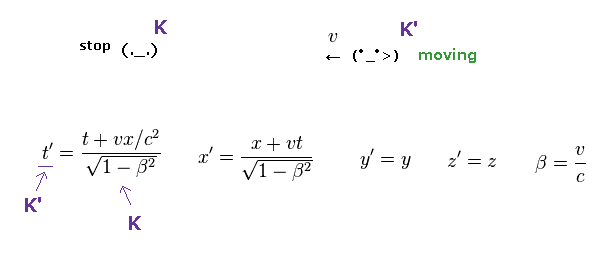K' frame is moving at v to the left relative to K frame.
So Lorentz transformation between K and K' frames become like Fig.13.

( t, x, y, z ) are time and space coordinates in K frame, and ( t', x', y', z' ) are in K' frame.
Change "v" into "-v" in Lorentz transformation of Fig.2.

## Why boxes on the upper side decrease from "5" to "3" ?

(Fig.14)   "5" boxes in K, "3" boxes in K' ← Paradox !Here we actually calculate each time and position using Lorentz transformation of Fig.13.
In Fig.14 left, the x coordinates ( x = 0, 3, 5 ) at the time of t = 0 are shown.

As I said in Fig.3, the state of Fig.14 left shows the collective states at different times in K' frame.
So we need to choose one same time ( for example, t' = 0 ) of K' frame to express the state of K' frame.

(Fig.15) Calculating each time and space in K' frame.Substituting each x-coordinate of x = 0, 3, 5 and t = 0 into Lorentz transformation of Fig.13, we can know the corresponding time and space in K' frame.

As shown in Fig.15 and Fig.16, the time (= t' ) on the left side ( x = 0 ) is earlier than that on the right side ( x = 5 ).

(Fig.16)   t' at x = 0 is earlier than t' at x = 5 !?So the state of Fig.14 left in K is collective states at different times of K' frame.
We need to make each time t' the same ( for example, t' = 0 ) to know how it looks from K' observer.

## How does it look from the viewpoint of K' observer ?

(Fig.17) If we look at the conveyor belt at t' = 0 in K' frame ...As I said in Fig.12, inner metallic frame is Lorentz contracted ( < l0 ), and each belt returns to its original length (= b0 ) in K' frame.
So Only "3" boxes can be put on the contracted upper side of this machine.

At t' > 0, the "4" and "5" boxes exist on the upper side, as shown in Fig.17.
So at the earlier time ( t' = 0 ), these two extra boxes exist on the right side along vertical inner frame.

## "Rigid" wires are bent complexly just by observer's movement ?

(Fig.18) Rigid wires are bent Only in K' frame !?If special relativity is right, we have to accept impossible situation like Fig.18 right.
As I said in Fig.16, the times in K' are different at different x coordinates.

If we try to reconstruct the state at the same time ( t' = 0 ) of all wires and boxes, this long wire becomes bent complexly.

The left part of this long wire (= 4 or 5 ) is straight along the horizontal direction.
But the right part of the same wire must be bent complexly, as shown in Fig.18 right.

## Rigid wire is "bent" conveniently to avoid paradox at detector ?

(Fig.19) Rigid wire can be bent in "convenient" way to avoid paradox in detected number ?If this rigid long wire can be bent obeying the time t' of special relativity, this system can avoid the paradox of different detected numbers ( 5 ← 3 ).

But of course, this is impossible, because the wire and box are rigid bodies, which must keep the same form.
This bent form of wire is very unnatural and unreal.

## One part is straght, and other parts are bent in the same wire !?

(Fig.20) Rigid long wire can be bent conveniently ?   Or special relativity is wrong.If special relativity is correct, the left part of this wire is straight horizontally.
And only middle part of this same wire must be bent complexly.

All parts contained in the same wire are the same kind of thing with respect to direction and motion.
So this weird situation of rigid wire, in which only one part is bent, is impossible. It means special relativity is wrong.

## We can change "future" state without touching it !?

(F-1)   Motion of the box 4 with wire in K frame.Here we explain about unreasonable phenomenon caused by special relativity.
In F-1, K frame observer is watching the movement of box 4 and its wire.

(F-2)   Rigid wire is "bent" just by simple observer's movement !?As I said in Fig.19, this rigid wire is bent from the viewpoint of K' observer.
Of course, this cannot happen in this real world, so special relativity contains fatal flaws in it.

In events happening at the same time in K' frame, the place on the left side shows the future event in K frame.
And the right side shows the past (= earlier ) event.

## The moment we insert new path, "future" changes ? ← Paradox !

(F-3)   We can change "future" state of the wire whithout touching it !? ← Paradox !Surprisingly, if Nature obeys special relativity, we can change future state of the wire without touching it !.
In F-3, we suddenly insert new path, which will carry the box upward ( NOT toward detector ) in the future.

This box has NOT arrived at this new path, so it doesn't know this existence at all.
In spite of this, the left side of wire becomes straight toward upper-left, just after inserting this path !

Because, if we insert this new path, this box is going upward without turining left in the future.
This means we can change the left side (= future state in K ) of this wire without thouching it !

So this relativistic world is completely unrealistic and false, considering various fatal paradoxes.

------------------------------------------------------------------------

### [ Rigid body behavior under the limit of light speed. ]

(F-4)   Small forces at different points at the same time. → long object moves simultaneously.Basically, the transmission speed of forces must obey light speed c ( "superluminal" is impossible ).
So when you accelerate a long object at the same time, you need to apply forces to the different points simultaneously, as shown in F-4.

If you apply the force only to one point of this object, it takes some time (= its total length / c ) to start to accelerate it.
As the number of points where force is applied increases, it takes less time to start to accelerate it.

In this case, the form and length of this rigid-body do NOT change in any places.
So this can happen under the rule of rigid-object.

### [ Only one obstacle can prevent the whole object from accelerating. ]

(F-5)   If a rigid path is inserted, the whole wire goes upward neglecting small other forces.In rigid body, any pieces of a long wire stick tightly to the nighboring ones through strong local potential energies.
So we cannot stretch or break any single point inside a rigid body.

When we suddenly insert a new path like F-3 and F-5, this rigid wire cannot accelerate to the left
So only one strong obstacle ( like this path ) determines the moving direction (= upward instead of left ) of this wire.

Because if only the left part of this wire moves to the left, some places of this long wire are stretched, which needs extremely strong forces ( from both sides ) overcoming local potential energy.

To accelerate a rigid body in some other directions, we have to remove any single resistance.
If a strong resistance is left, all other small forces become meaningless.

As a result, if special relativity is correct, we have to accept paradoxical phenomenon in which the future state can be changed without touching it like F-3. So relativistic world is false.

------------------------------------------------------------------------

## Paradox of electromagnetism is the same pattern.

(Fig.21) Charge and current paradox is the same pattern.This paradox in electromegnetic fields is the same pattern as this moving conveyor belts with long wires.

We consider moving boxes as electric current of negative charges.
And the same amount of positive charges at rest are included on each side of the frame.

(Fig.22) Negative charges decreases from "5" to "3" ← Paradox.As shown on this page, using Lorentz transformation of charge density (= ρ ) and current (= J ), we can know the electric current on the upper side changes into positive in K' frame from neutral in K.

Current loop contains two directions (= horizontal and vertical ).
Relativistic electromagnetism can NOT control two different directions.

In Fig.22 left, an external negative charge is at rest in K frame, but this charge is attracted toward current in K', because neutral currents in K changes to positive in K'.
This is clearly a fatal paradox, and proves special relativity is wrong.

## Special relativity is false → Inflation, black hole, SUSY are all wrong.

(Fig.23) Special relativity is false → general relativity is false. Why fantasy theories must be believed ?General relativity contains inertial frame of special relativity as a part of it.
So if special relativity is false, general relativity is false, too.

As a result, Inflation, black hole and SUSY are all false, because they are all based on relativity.

In fact, even if we continue studying about these fictitious concepts such as early universe and black hole, our science does NOT make progress at all, which means they are wasting much time in these fantasy theories.

These concepts including SUSY and string theory (= 10 dimensions ) cannot be confirmed directly, and useless, forever.
So it is just equal to saying these theories are unreal.

## Paradox of forces is caused by relativistic momentum.

(Fig.24) Two equal forces Fy are in equilibrium.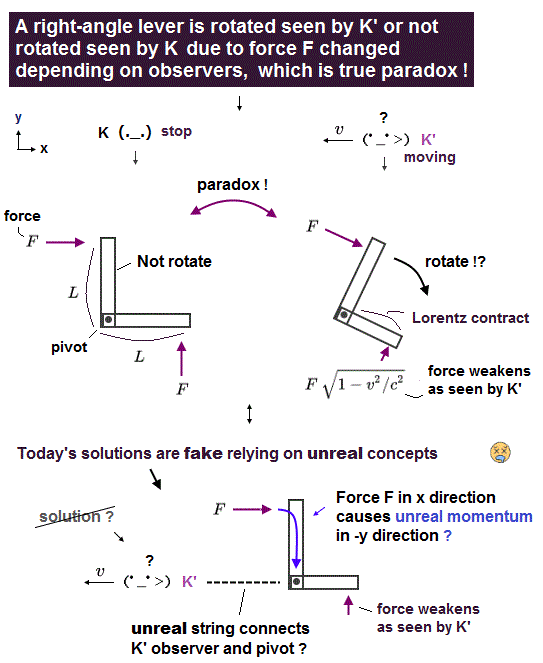The upper paradoxes such as electromagnetism and conveyor belt are based directly on Lorentz transformation itself.

On the other hand, this paradox in force transformation is based on the new introduction of relativistic momentum and energy.
So this is independent from the upper cases.

In Fig.24, two equal forces Fy are applied to the stationary lever in K frame (= observer at rest ).
This lever has two equal arms on both sides of a fulcrum, so it is in balance, NOT rotate in K frame.

One of these forces (= Fig.24 left ) is at rest, and another force ( source ) is moving at velocity "v" in x direction.

## Lever rotates Only in K' frame. ← Paradox !

(Fig.25) Lever rotates from the viewpoint of moving observer (= K' ) ?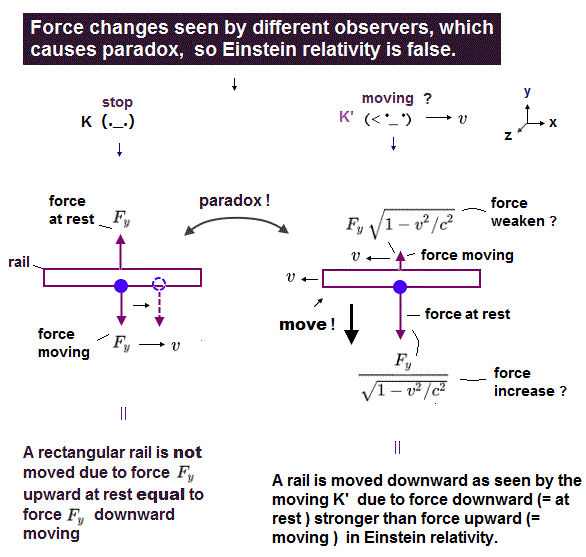When the observer is moving at "v" in x direction (= K' ), this lever and left force start to move in the opposite direction.
As a result, this force (= Fig.25 left ) is reduced, accodring to special relativity

On the other hand, right force looks stationary in K' frame, it is increased by observer's movement.
As a result, this lever rotates clockwise only in K' frame !

This unreasonable transformation of force Fy is one of causes of right angle lever paradox.
Of course, this paradox has NO solutions, which means relativistic momentum and energy are wrong.

## General relativity reveals "twin paradox".

(Fig.26) Twin paradox in the two identical satellites.As shown in Lorentz transformation, each time and space coordinate in one inertial frame corresponds to that of another frame, independently.

So, we cannot prove twin paradox within special relativity.
But the "time dilation" experiments using atomic clock ( in moving objects ) are very doubtful due to twin paradox.

To prove twin paradox by going back to the same place, we have to depend on general relativity.
Because general relativity adopts a different kind of concept such as central gravitational force.

And as shown on this page, when the two identical satellites are moving in the opposite directions on the same orbit around the earth, their clock times clearly show paradox.

Seen from one satallite (= A ), the clock of another satellite (= B ) ticks slower.
But seen from "B" satellite, the clock of "A" satellite ticks slower. So which clock is slower ?

General relativity can make these two satellites meet each other, using gravity.
So twin paradox is revealed with the help of general relativity.

## Supplement to paradox in electric fields.

(Fig.27) Negative charge is attracted toward wire ONLY in S' frame ?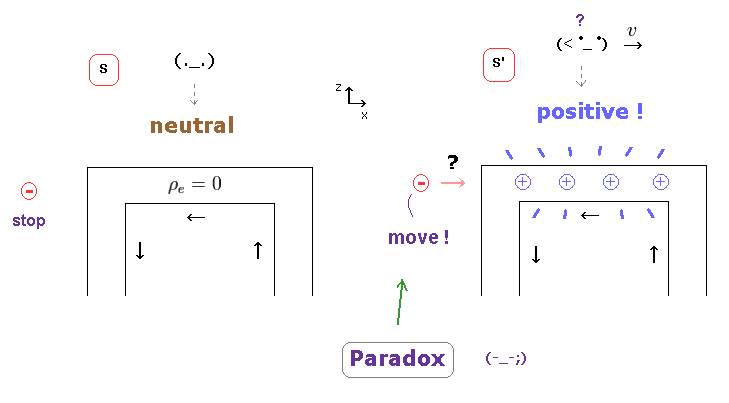On this page, we show charge densities included in the upper ( or lower ) wire changes by oberver's movement.
In this section, we review the transformation of charge and electric field in different inertial frames.

## Lorentz transformation of charge and current densities

(Eq.1) The universal relation between charge (= ρ ) and current (= J ).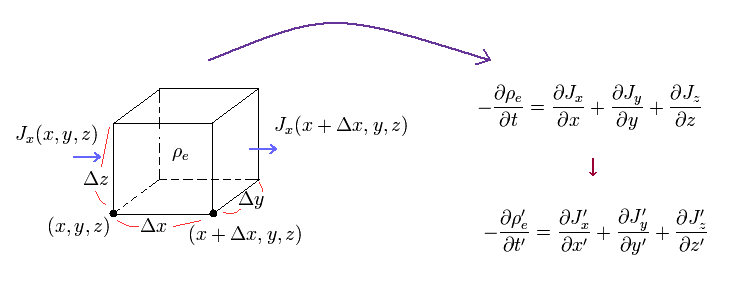As shown on this page, the increase in charge density (= ρ ) must be equal to the gradient of current density (= J ) in all reference frames.
Because it is impossible that "charges" suddenly disappear somewhere.

(Eq.2) Lorentz transformation of charge (= ρ ) and current (= J ).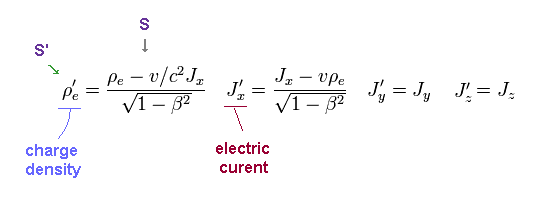To satisfy the relation of Eq.1, the charge density (= ρ ) and current density (= J ) must transform like Eq.2 under Lorentz transformation.
Apostrophe' denotes S' frame, which is moving in x direction at v, relative to S frame.

## Total charge Q is conserved in different frames.

(Fig.28) Total charge Q = Q' in S and S' frames.Using Lorentz transformation of Eq.2, we can know the total charge Q is always conserved in different inertial frames.
In Fig.28 left, a cube of charge density (= ρ ) is at rest in S frame.

The length, width, heigh of this cube is supposed to be x, y, z.

(Fig.29)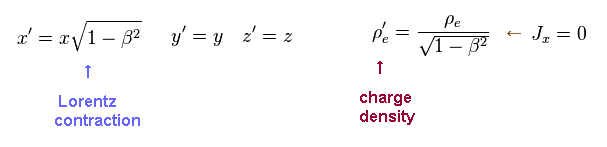Seen from S' observer moving in x direction at v, only the length of moving direction (= x' ) is Lorentz-contracted.
And using Eq.2, charge density (= ρ' ) is increased, as shown in Fig.29.

(Fig.30) Total charge Q does NOT change.Total charge Q is given by the charge density (= ρ ) × the volume (= xyz ) of this cube.
From Fig.29, you can understand the total charge Q does NOT change in S' frame ( Q = Q' ).

This means the total charge "e" of an electron and a proton is the constant value, independent from observer's motion.

## Electrinc field and force in moving direction do NOT change.

(Fig.31) Gauss law and transformation of electric field Ex.When some charge is enclosed by the surface S, this total charge is given by the surface integral of electric field over S.
According to special relativity, the electric field E and Newtonian force F in the moving direction ( in this case, Ex ) do NOT change under Lorentz transformation.

In Fig.31, there is no contradiction between the electric and Newtonian force acting on an external charge (= + ).
Because they are the same in different frames.

From Fig.30 and Fig.31, we can know Gauss law, which defines the relation of electric field and charge, is always effective even when the charge is moving.

(Eq.3) Lorentz transformation of electromagnetic fields.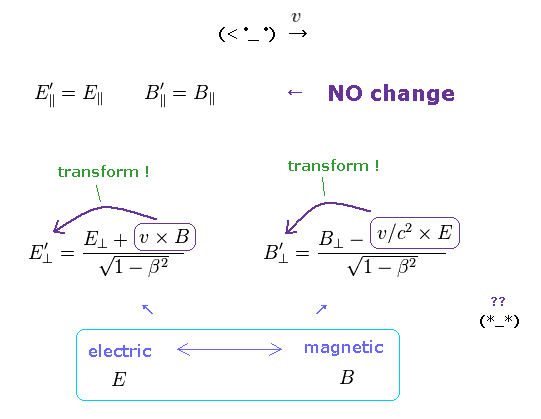As shown on this site and this site (p.91), Lorentz transformation of E, B fields become like Eq.3.
This transformation is indispensable to unite special relativity and Maxwell equation.

(Fig.32) Lorentz transformation.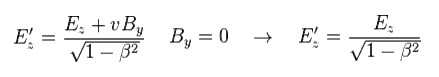Using Lorentz transformation of Fig.32, we can prove Gauss law is effective also in y and z directions.
As shown in Fig.33, the charge density (= ρ ) in x-y plane is increased in S' frame.

(Fig.33) Gauss law is always valid in S and S' frames.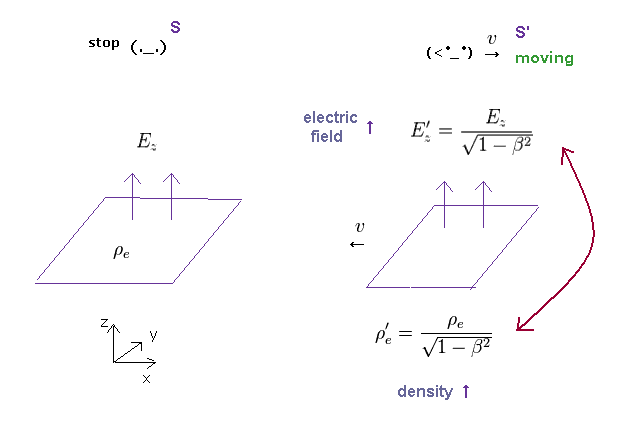In proportion to this increase in charge density, the electric field Ez ( or Ey ) is increased, too.
As a result, the surface integral of electric field becomes equal to enclosed total charge in any reference frames (= Gauss law ).

## Coulomb law is effective in all directions in different frames.

(Fig.34) Surface intagrals over different areas give the same charge Q.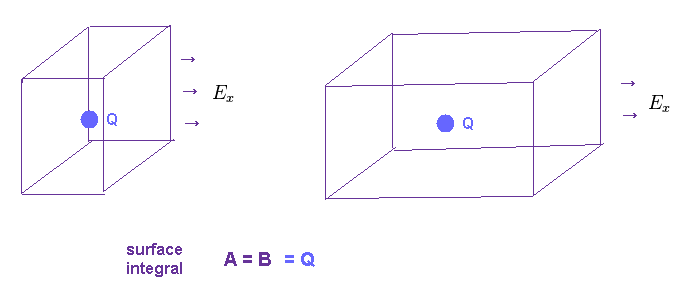Of course, if the amount of total charge is the same, any different surface integrals of electric field around the charge must give the same amount of charge.

If the strengths of Ex and Ey change differently under the same total charge, these surface integrals give different values, which means wrong.
( In this case, the surface integrals of Fig.34 left and right over different forms of cubes give different Q. )

(Fig.35)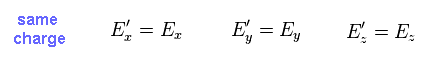So, if the total charge included is the same, the strengths of electric field in all directions do NOT change under Lorentz transformation.

And fatal paradox of relativistic electromagnetism appears, as shown in Fig.27 and Fig.36.

(Fig.36) Electric field E'x is zero, but E'z is NOT zero. ← Paradox !   strange charges.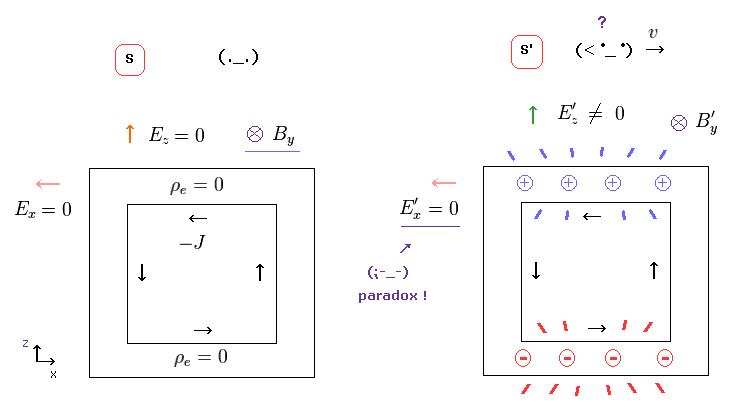### [ Which is true ?   Muon's lifetime is longer or shorter ?   ← Twin paradox ! ]

(Fig.37)   Observer on the earth (= A ) and one moving with muon (= B ).Though it is said that the time dilation can be confirmed (← ? ) by muon's longer lifetime, this time dilation is self-contradictory.   See also this and this.

In Fig.37, from the perspective of an observer on the earth (= A ), the muon is moving, so muon's clock is time-dilated. See this site.
Suppose muon's lifetime is "4" seconds, while 4 seconds have elapsed in muon's frame, 8 seconds have elapsed on the earth.

So in the above case, the muon's clock is time-dilated, and its lifetime looks longer (= Fig.37 left ).
But from the perspective of the observer moving with muon (= B ), the clock on the earth is time-dilated.

Because, the earth is moving and the muon is at rest in muon's frame (= Fig.37 right ).
As a result, while 4 seconds elapse in muon's frame, only 2 seconds elapse on the earth. (= Muon's lifetime is shorter ! )

This is clearly a twin paradox, and shows the experimental facts of "time-dilation" is doubtful, and wrong.
The same thing can be said about the GPS time dilation (← far-fetched interpretation ).

## Time dilation in atomic clocks is doubtful.

(Fig.38) Light source is moving at "v", and emitting light with frequency f'.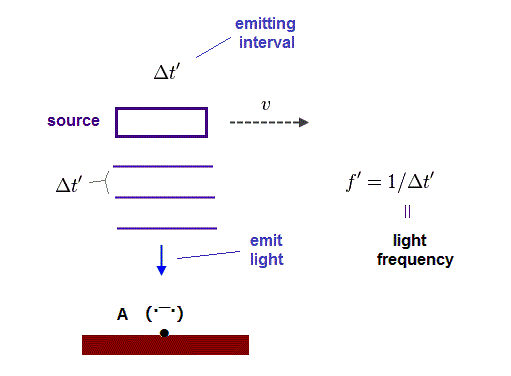It is said that time dilation was confirmed using airplanes, these experiments depended on many artificial corrections.   Basically when a plane comes back to the original place, it causes twin paradox.

In these sites ( this and this ), they argue that atomic clocks using emitted light's frequency confirmed time dilation in moving objects.   But due to twin paradox, the time dilation becomes opposite, which means these confirmations are doubtful.

In Fig.38, the light source is moving to the right at a velocity of "v", and emitting light with a frequency of f'.   Time period between wave crests in the source rest frame is (= Δ t' ).

Here the source's time is t' and the observer's time is t.

## Time dilation in moving source ?

(Fig.39) Clock in moving source is time-dilated ?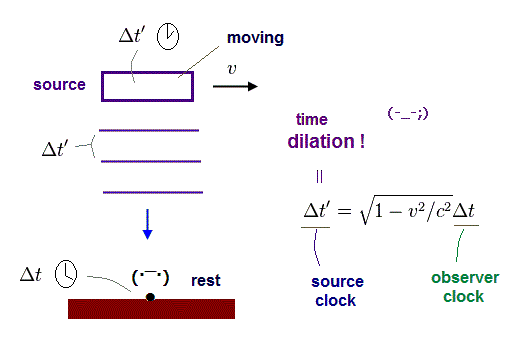According to special relativity, the moving clock runs more slowly than that at rest.
So, while Δ t' elapse in the source's clock, more time (= Δ t > Δ t' ) elapse in the observer's clock.

(Fig.40) Observed light frequency decreases ?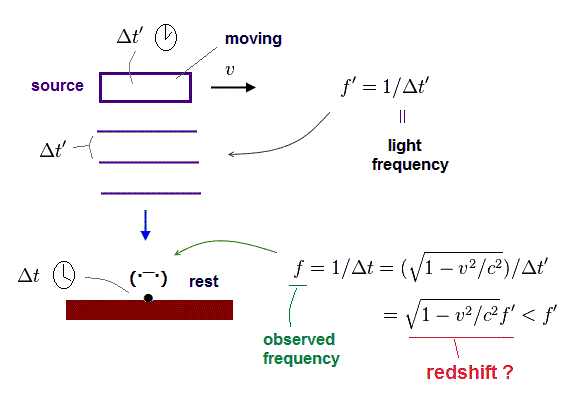As a result, they argue that the observed light frequency decreases (= f < f' ). This is called "redshift"
Because of the time dilation, more time elapse in rest frame during one light wavelength ( Δ t > Δ t' ).

## But in the source's rest frame, the observer is moving !

(Fig.41) Source is at rest, and the observer is moving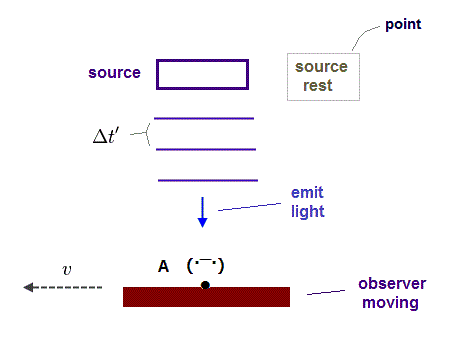But from the perspective of light source (= rest frame of source ), the observer is moving in the opposite direction.

## Time dilation becomes opposite.

(Fig.42) Observer's clock ticks more slowly in this case ?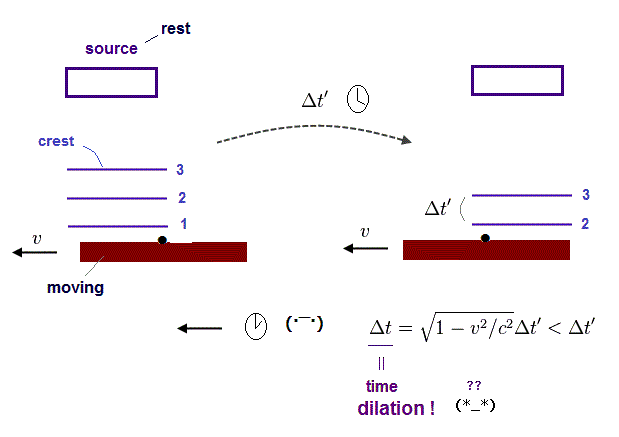As a result, the observer's clock ticks more slowly than the source's clock in this frame.
Because the observer is moving, and the source is at rest.

## Observed light frequency increases !? ← Paradox !

(Fig.43) Observed light frequency (= f ) "increases" in the source's rest frame ?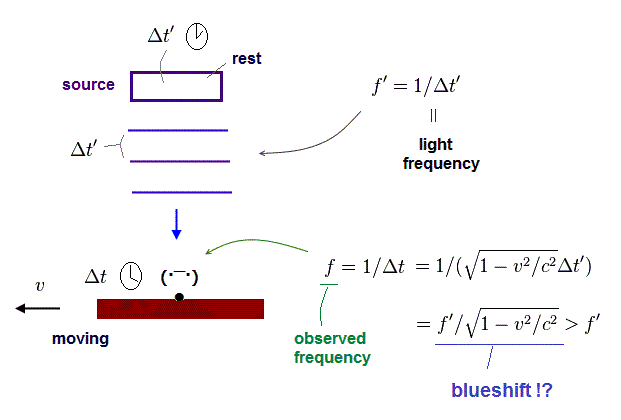Due to time dilation in observer's clock, the observed light frequency increases (= blueshift ) in this case.
This is clearly inconsistent with "redshift" in Fig.40.

## So, time dilation using atomic clock is very doubtful.

(Fig.44) Which is true ?   Redshift or blueshift ?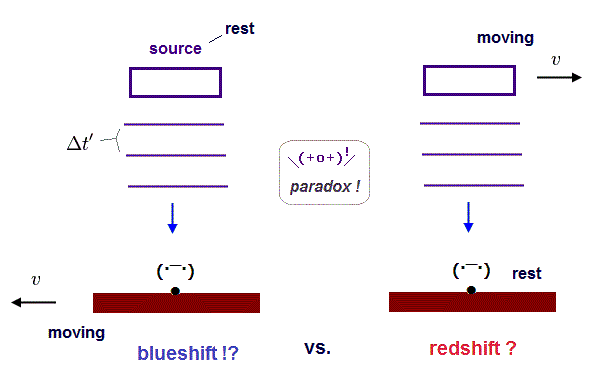As you see, we don't know which will happen, redshift or blueshift.
This is clearly paradox, and shows time dilation using atomic clock (= GPS, transverse Doppler ) is very doubtful and wrong.

In fact, the right answer is "blueshift", different from the current standard's claim, if special relativity is right.   Because when the light source is moving, the light is emitted "twisted", causing blueshift.

Redshift is caused by the "medium-Doppler shift" (= classical ), as is explained on this page.
So the interpretation in GPS and transverse Doppler by atomic clocks contradicts relativity.2015/1/6 updated. Feel free to link to this site.